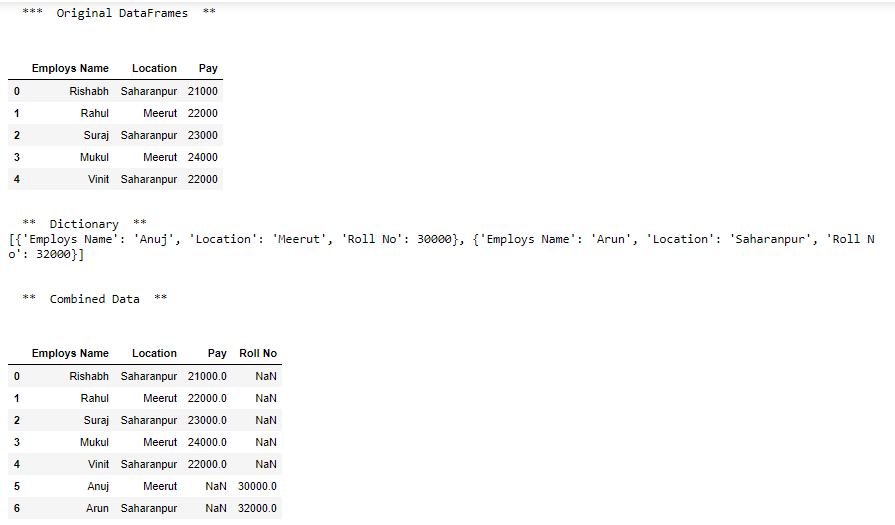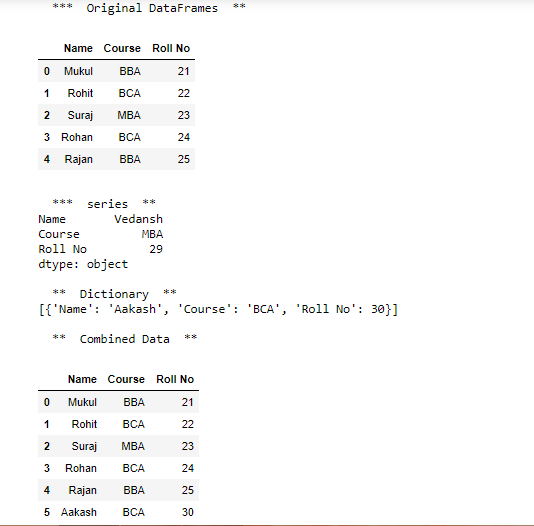# Append list of dictionary and series to a existing Pandas DataFrame in Python

In this article, we will discuss how values from a list of dictionaries or Pandas Series can be appended to an already existing pandas dataframe. For this purpose append() function of pandas, the module is sufficient.

Syntax: DataFrame.append(other, ignore_index=False, verify_integrity=False, sort=None)

Parameters :
other : DataFrame or Series/dict-like object, or list of these
ignore_index : If True, do not use the index labels.
verify_integrity : If True, raise ValueError on creating index with duplicates.
sort : Sort columns if the columns of self and other are not aligned. The default sorting is deprecated and will change to not-sorting in a future version of pandas. Explicitly pass sort=True to silence the warning and sort. Explicitly pass sort=False to silence the warning and not sort.

Returns: appended : DataFrame

Approach

• Import module
• Create data frame or series
• Create a list with dictionaries
• Append this list to existing data frame or series

Example 1:

## Python

 `# import pandas` `import` `pandas as pd`   `# create dataframe` `df ``=` `pd.DataFrame({` `    ``'Employs Name'``: [``'Rishabh'``, ``'Rahul'``, ``'Suraj'``, ``'Mukul'``, ``'Vinit'``],` `    ``'Location'``: [``'Saharanpur'``, ``'Meerut'``, ``'Saharanpur'``, ``'Meerut'``, ``'Saharanpur'``],` `    ``'Pay'``: [``21000``, ``22000``, ``23000``, ``24000``, ``22000``]})`   `# print dataframe` `print``(``"\n  ***  Original DataFrames  **  \n"``)` `print``(df)`     `# create dictionaries` `dicts ``=` `[{``'Employs Name'``: ``'Anuj'``, ``'Location'``: ``'Meerut'``, ``'Roll No'``: ``30000``},` `         ``{``'Employs Name'``: ``'Arun'``, ``'Location'``: ``'Saharanpur'``, ``'Roll No'``: ``32000``}]`   `# print dictionaries` `print``(``"\n  **  Dictionary  ** "``)` `print``(dicts)`     `# combined data` `df ``=` `df.append(dicts, ignore_index``=``True``, sort``=``False``)`   `# print combined dataframe` `print``(``"\n\n  **  Combined Data  **\n"``)` `print``(df)`

Output:Example 2:

## Python

 `# import pandas` `import` `pandas as pd`   `# create dataframe` `df ``=` `pd.DataFrame({` `    ``'Name'``: [``'Mukul'``, ``'Rohit'``, ``'Suraj'``, ``'Rohan'``, ``'Rajan'``],` `    ``'Course'``: [``'BBA'``, ``'BCA'``, ``'MBA'``, ``'BCA'``, ``'BBA'``],` `    ``'Roll No'``: [``21``, ``22``, ``23``, ``24``, ``25``]})`   `# print dataframe` `print``(``"\n  ***  Original DataFrames  ** "``)` `display(df)`     `# create series` `s6 ``=` `pd.Series([``'Vedansh'``, ``'MBA'``, ``29``], index``=``[``'Name'``, ``'Course'``, ``'Roll No'``])`   `# print series` `print``(``"\n  ***  series  ** "``)` `print``(s6)`   `# create dictionaries` `dicts ``=` `[{``'Name'``: ``'Aakash'``, ``'Course'``: ``'BCA'``, ``'Roll No'``: ``30``}]`   `# print dictionaries` `print``(``"\n  **  Dictionary  ** "``)` `print``(dicts)`     `# combined data` `df ``=` `df.append(dicts, ignore_index``=``True``, sort``=``False``)` `print``(``"\n  **  Combined Data  **"``)` `display(df)`

Output:Whether you're preparing for your first job interview or aiming to upskill in this ever-evolving tech landscape, GeeksforGeeks Courses are your key to success. We provide top-quality content at affordable prices, all geared towards accelerating your growth in a time-bound manner. Join the millions we've already empowered, and we're here to do the same for you. Don't miss out - check it out now!

Previous
Next